# RS Aggarwal Solutions for Class 12 Maths Chapter 11: Applications of Derivatives Exercise 11F

Solved examples based on finding the maximum and minimum value and examples related to volume and area of solids are discussed under this exercise. Students can get a clear idea about the steps to be followed in solving exercise wise problems from the set of examples. The solutions are prepared by faculty at BYJU’S with the aim of helping students perform well in the board exam. RS Aggarwal Solutions Class 12 Maths Chapter 11 Applications of Derivatives Exercise 11F PDF links are given here for free download.

## RS Aggarwal Solutions for Class 12 Maths Chapter 11: Applications of Derivatives Exercise 11F Download PDF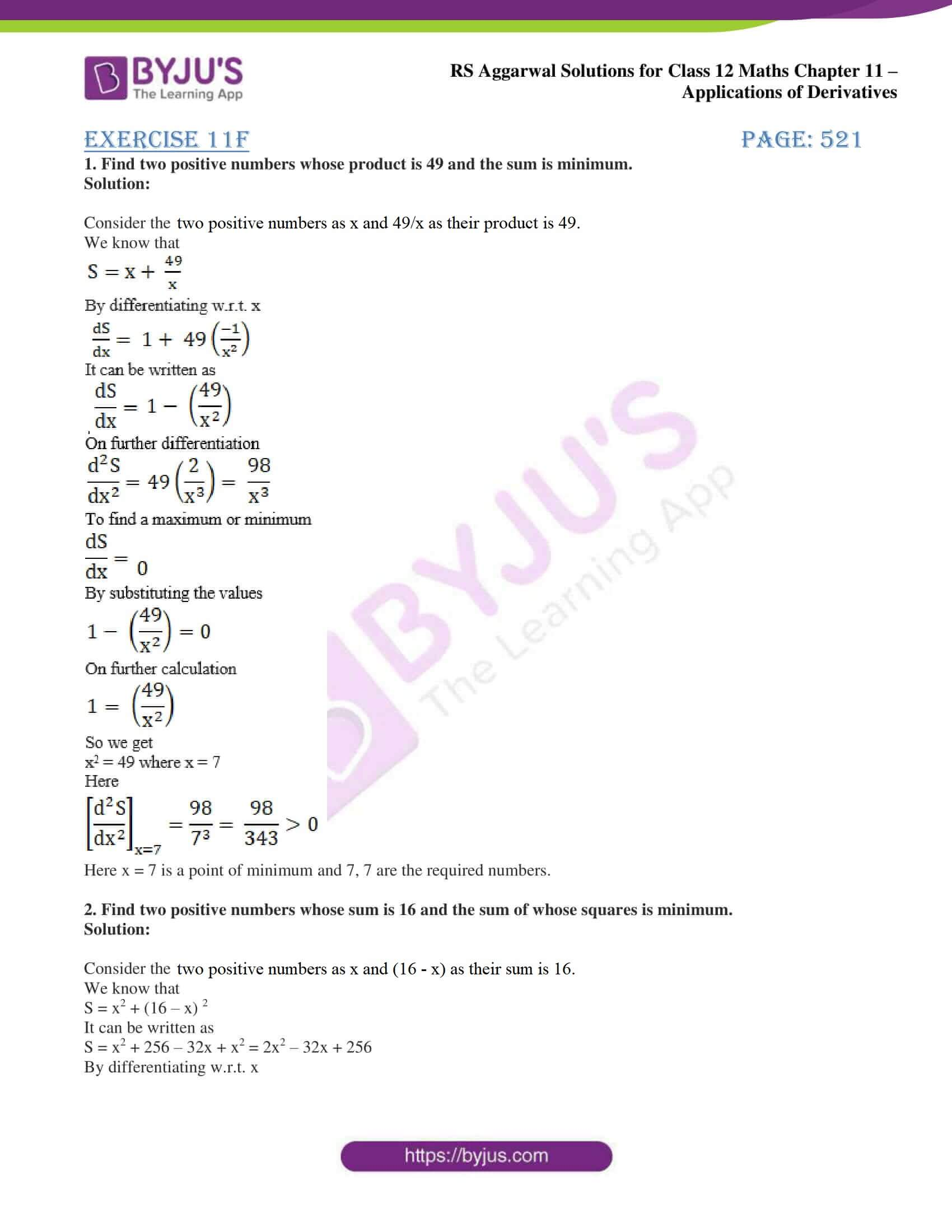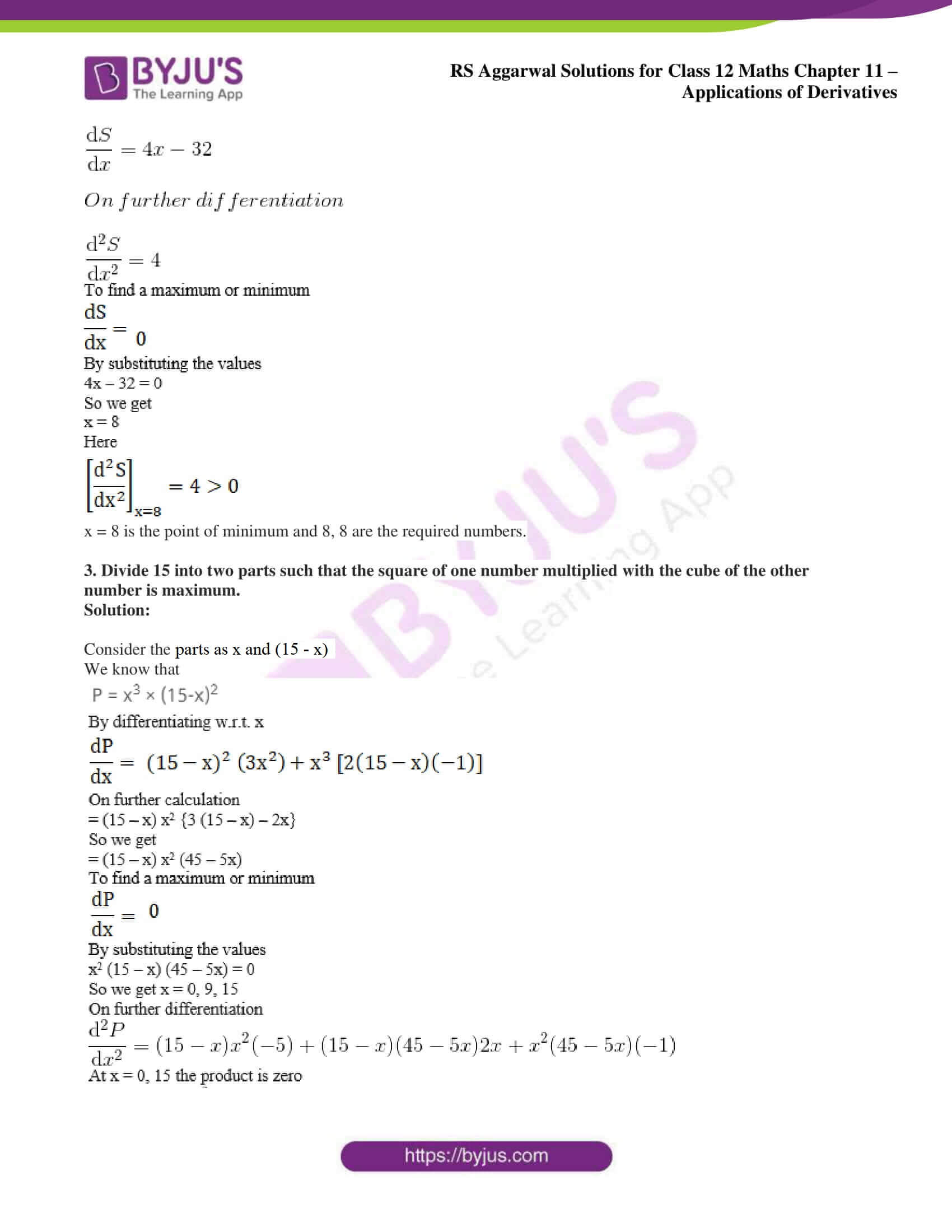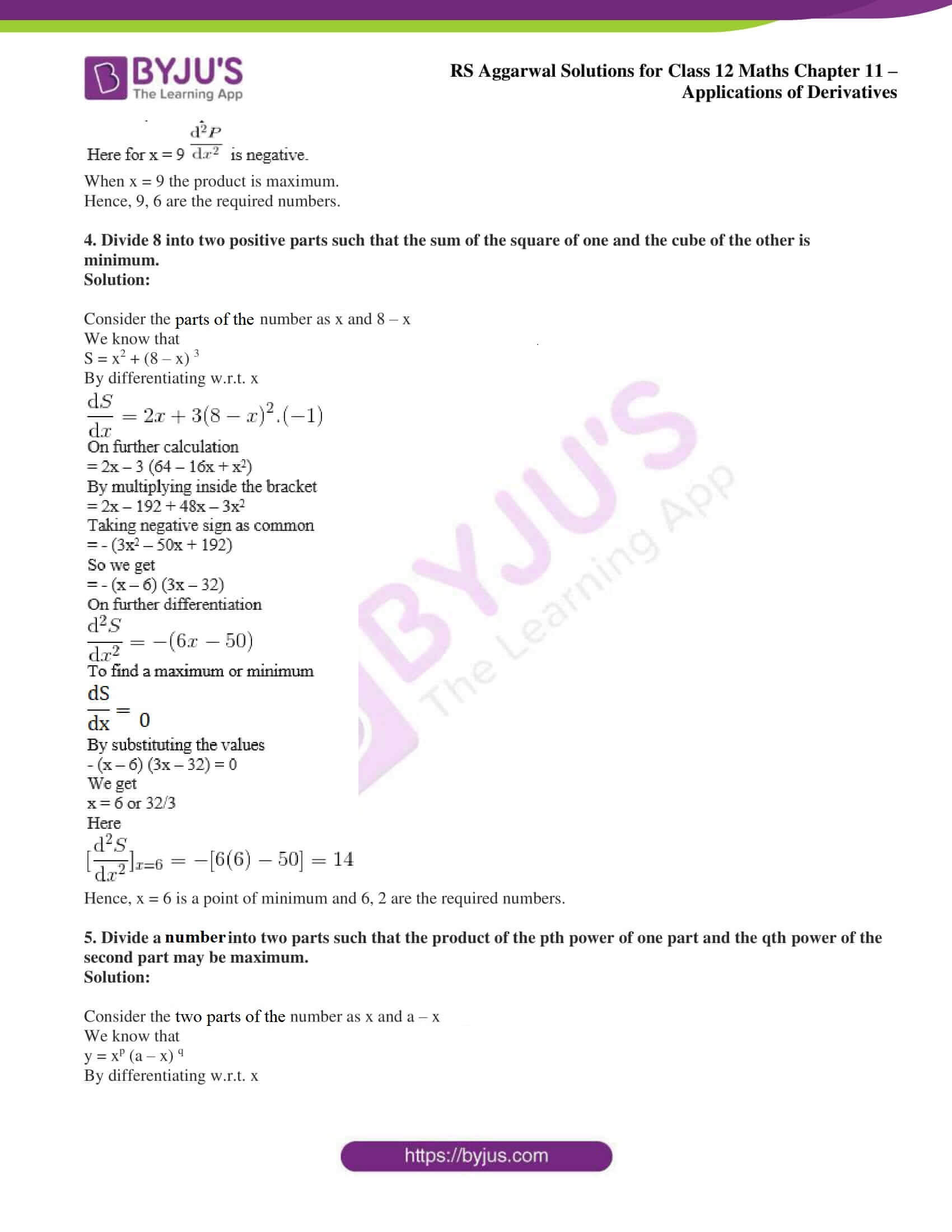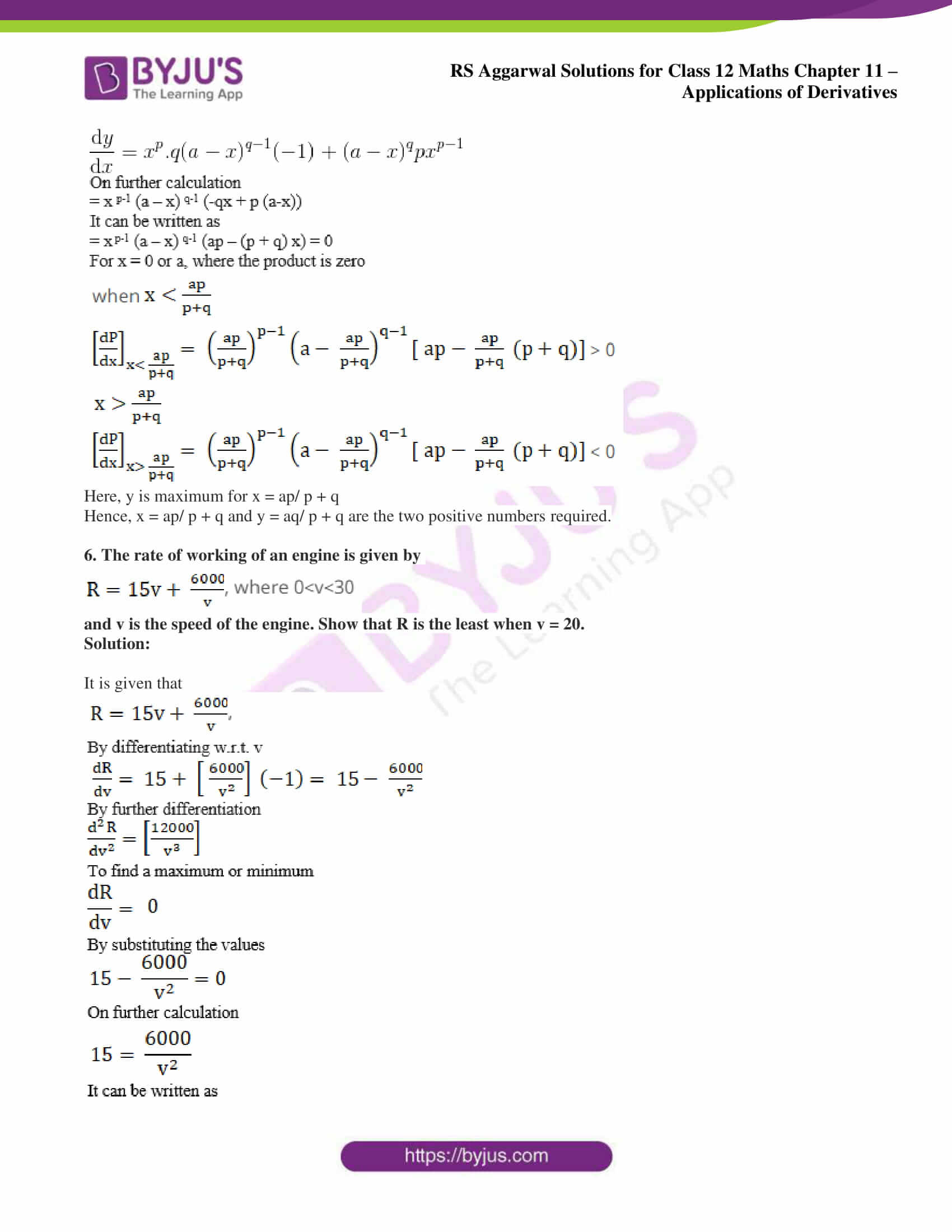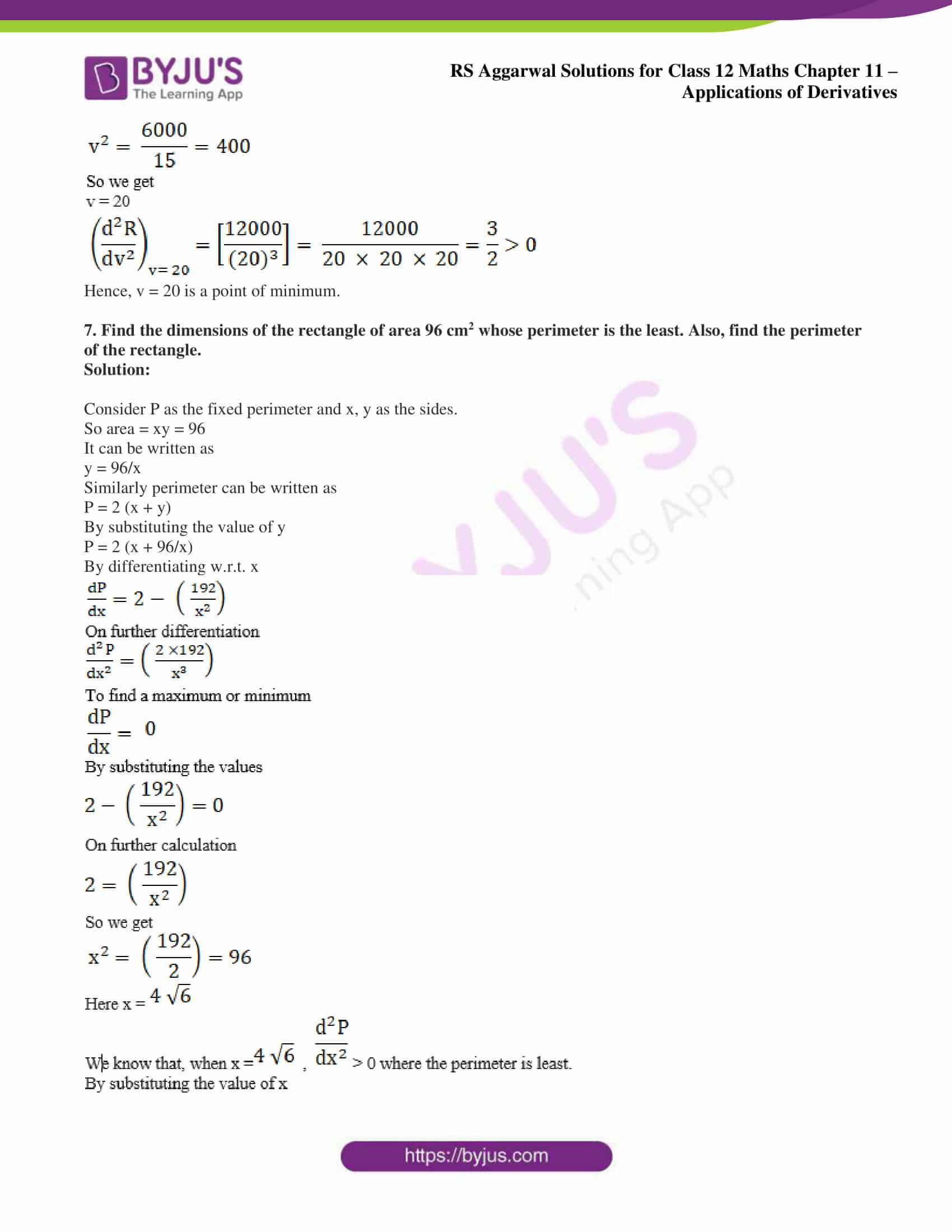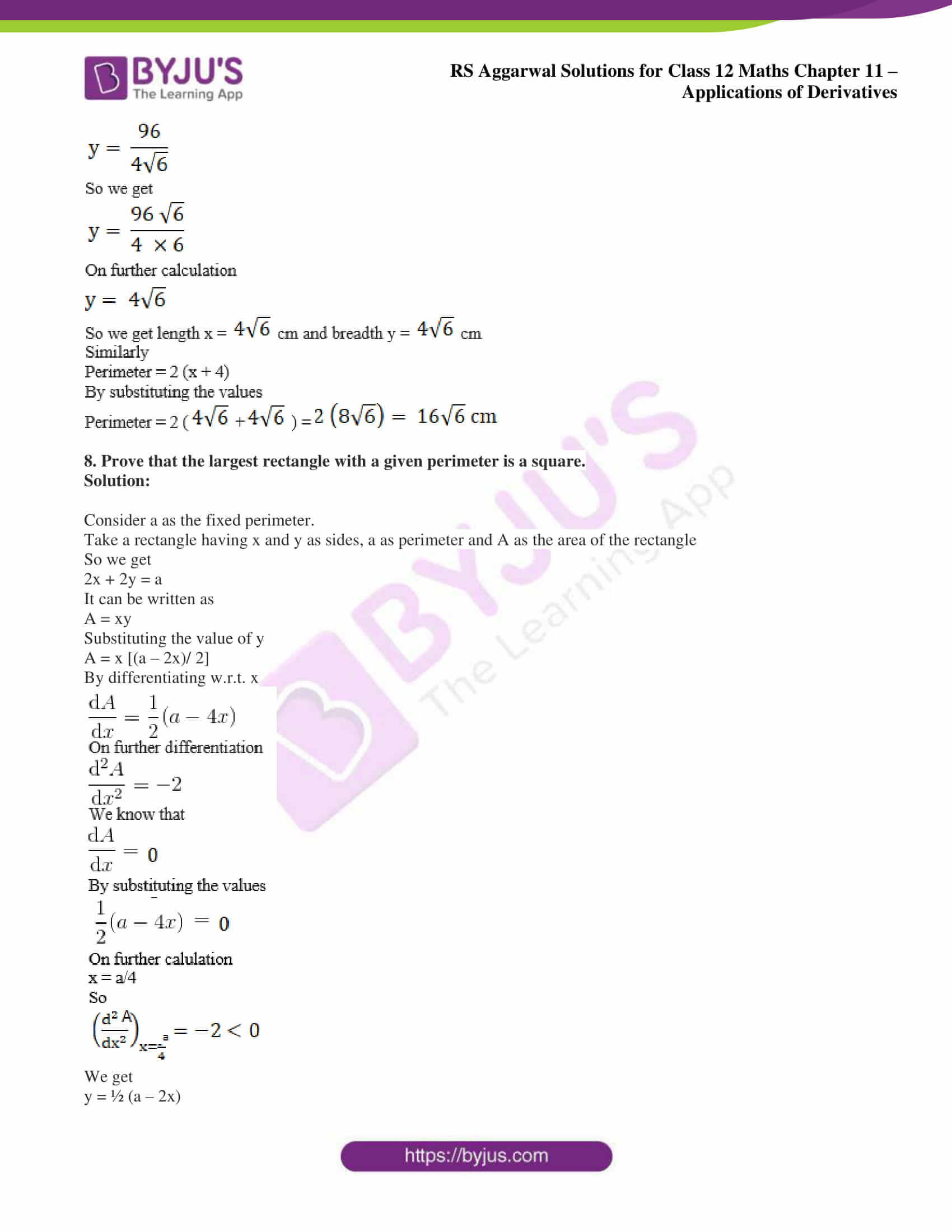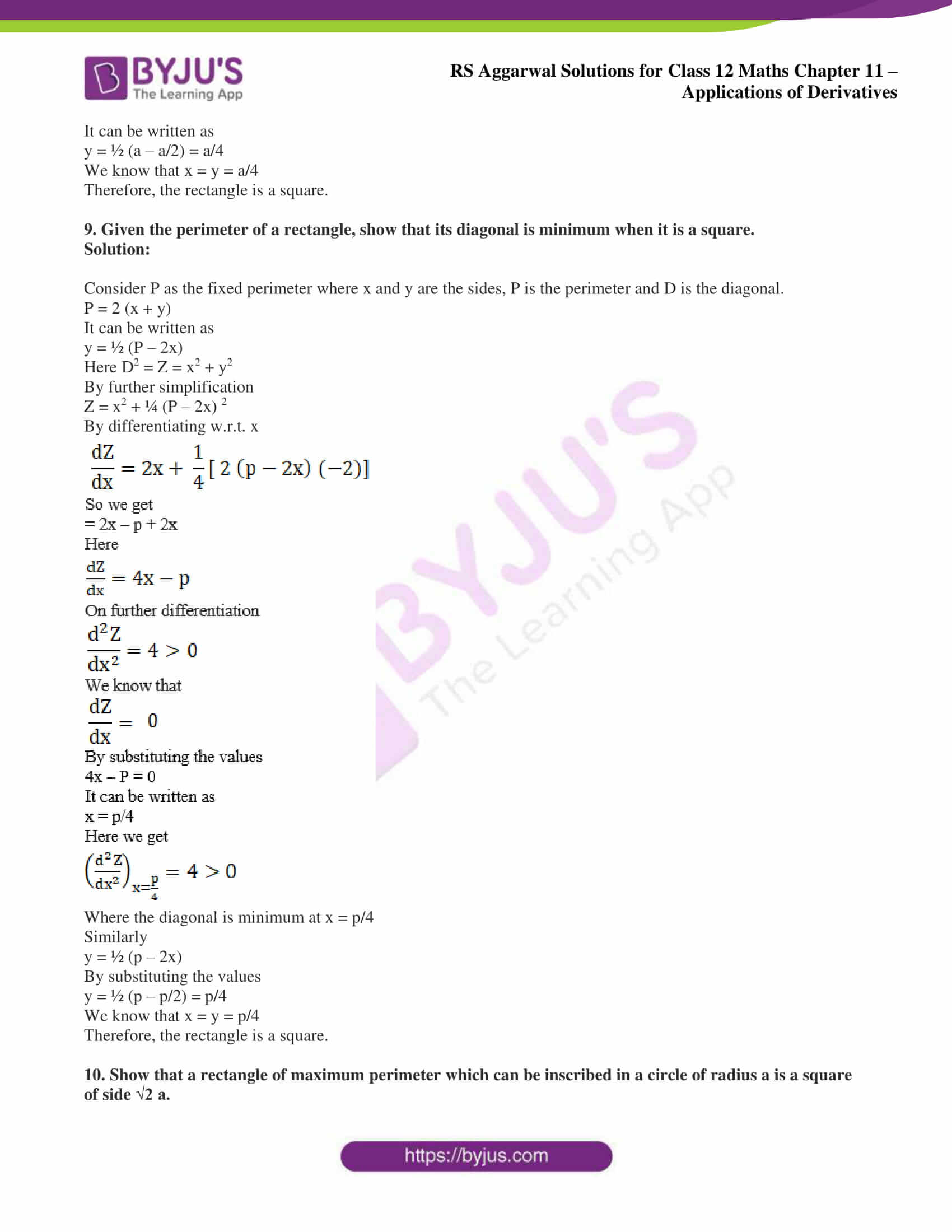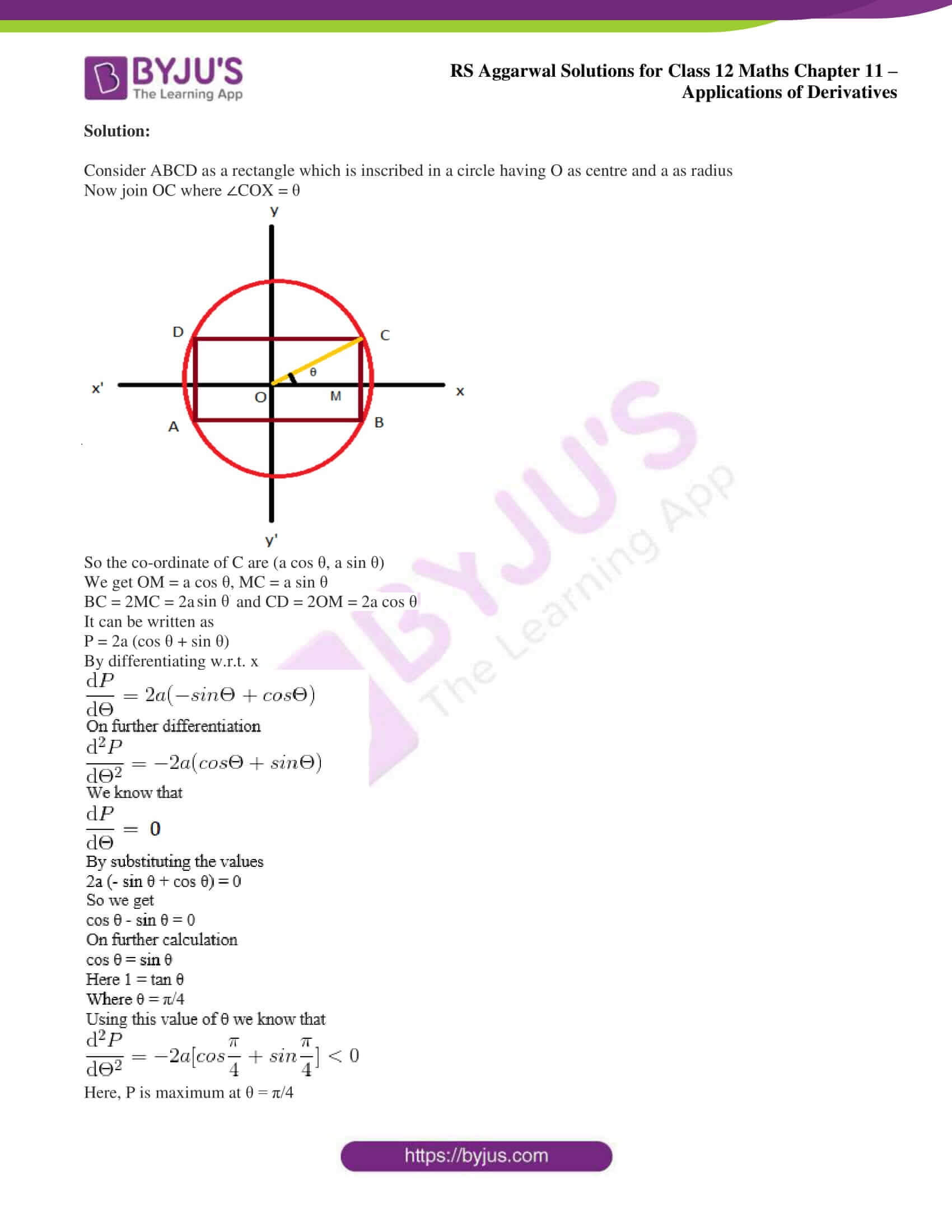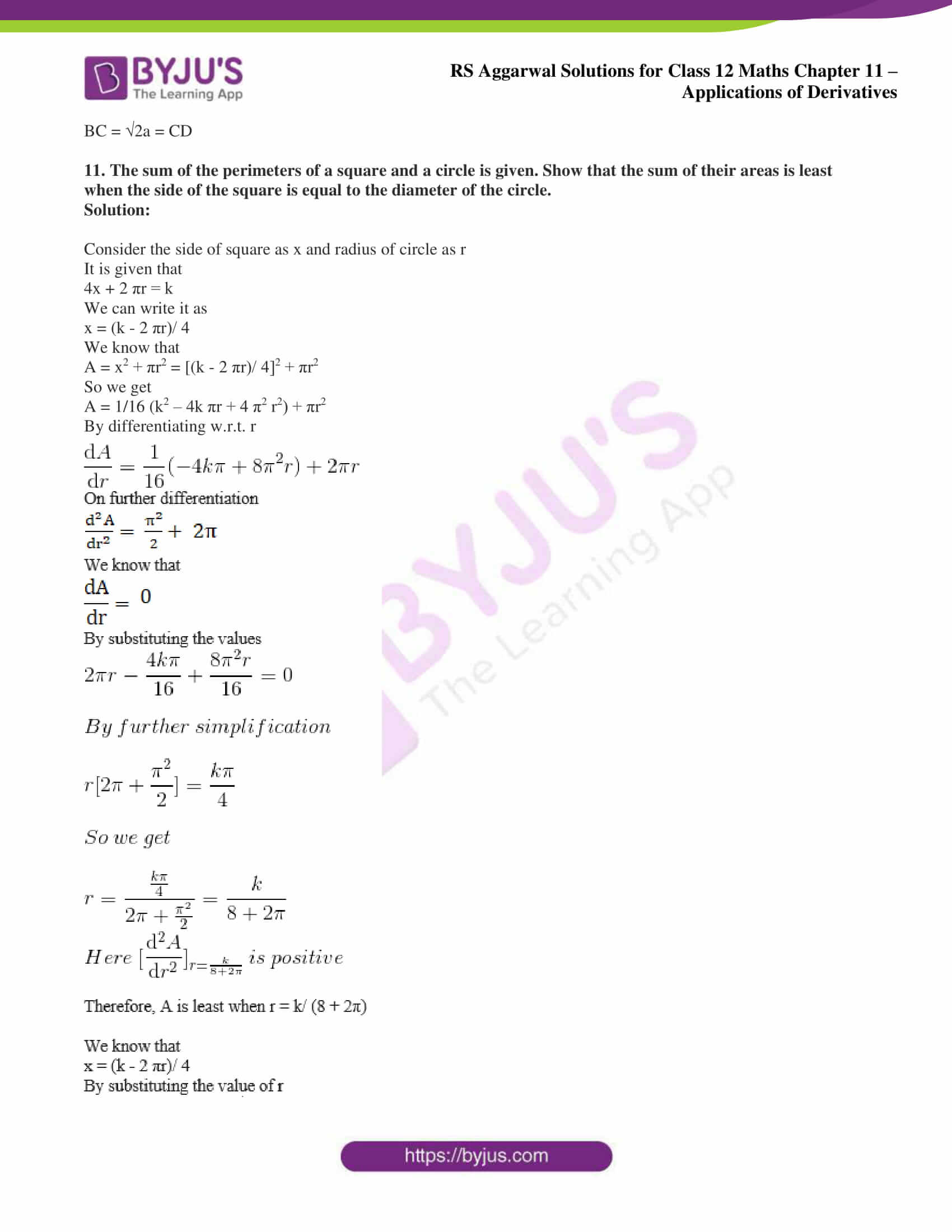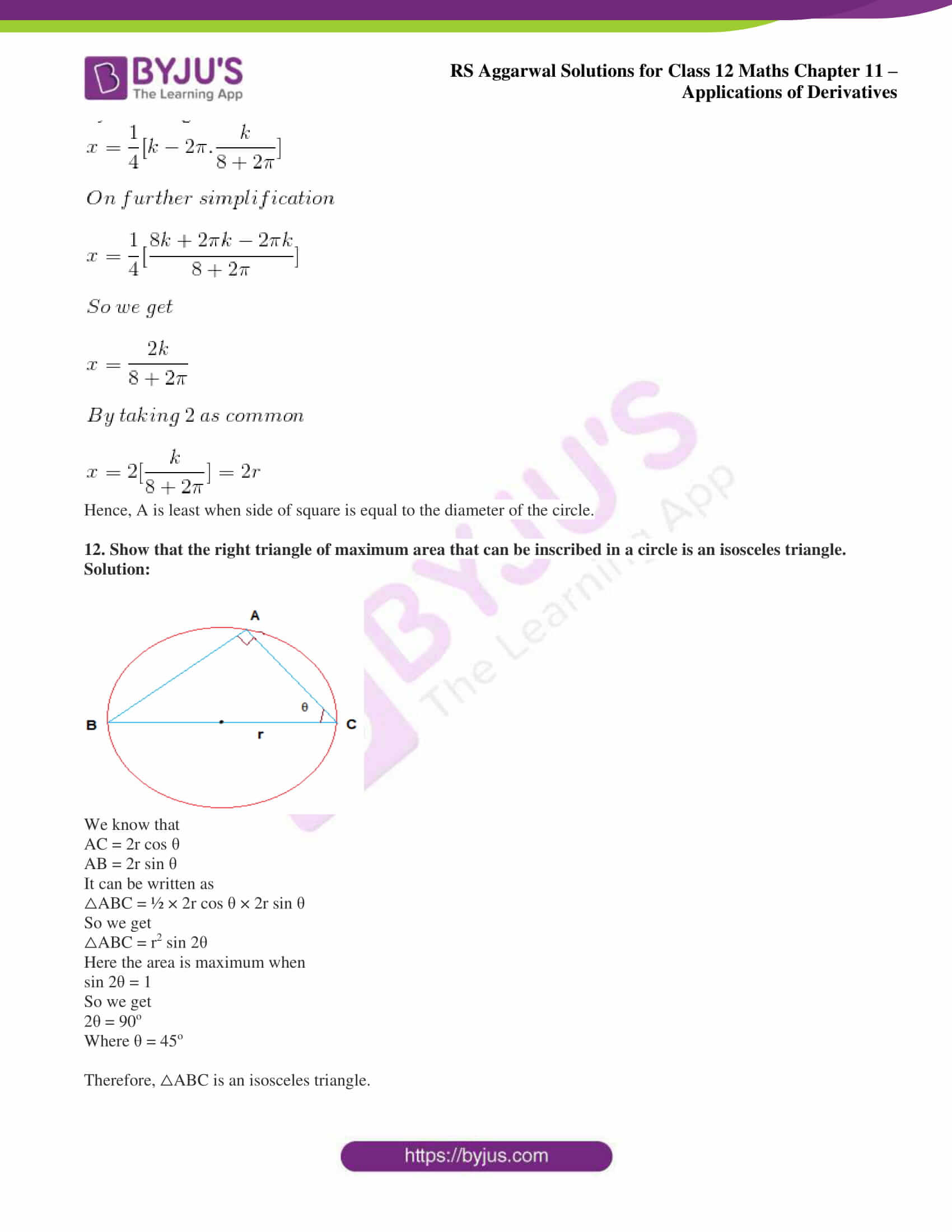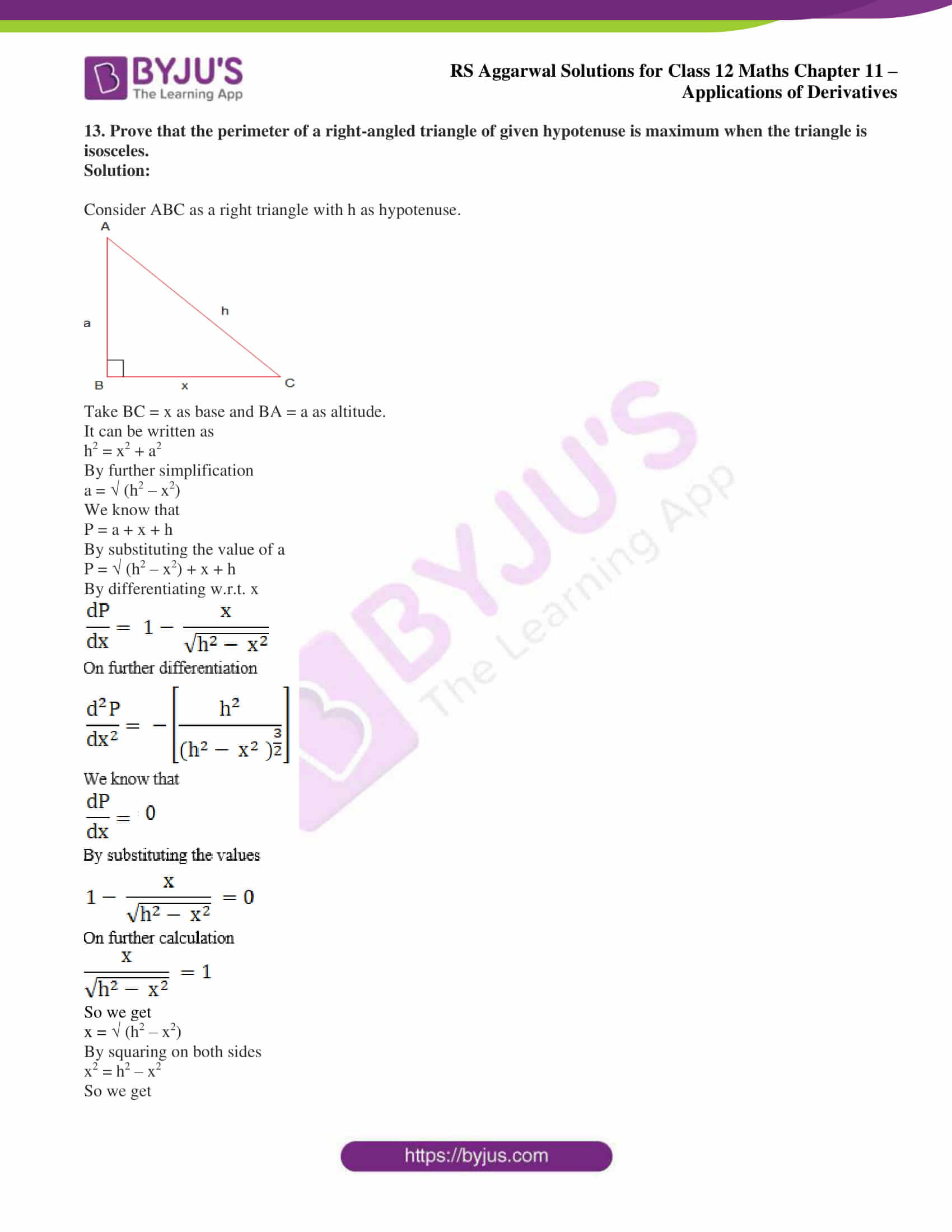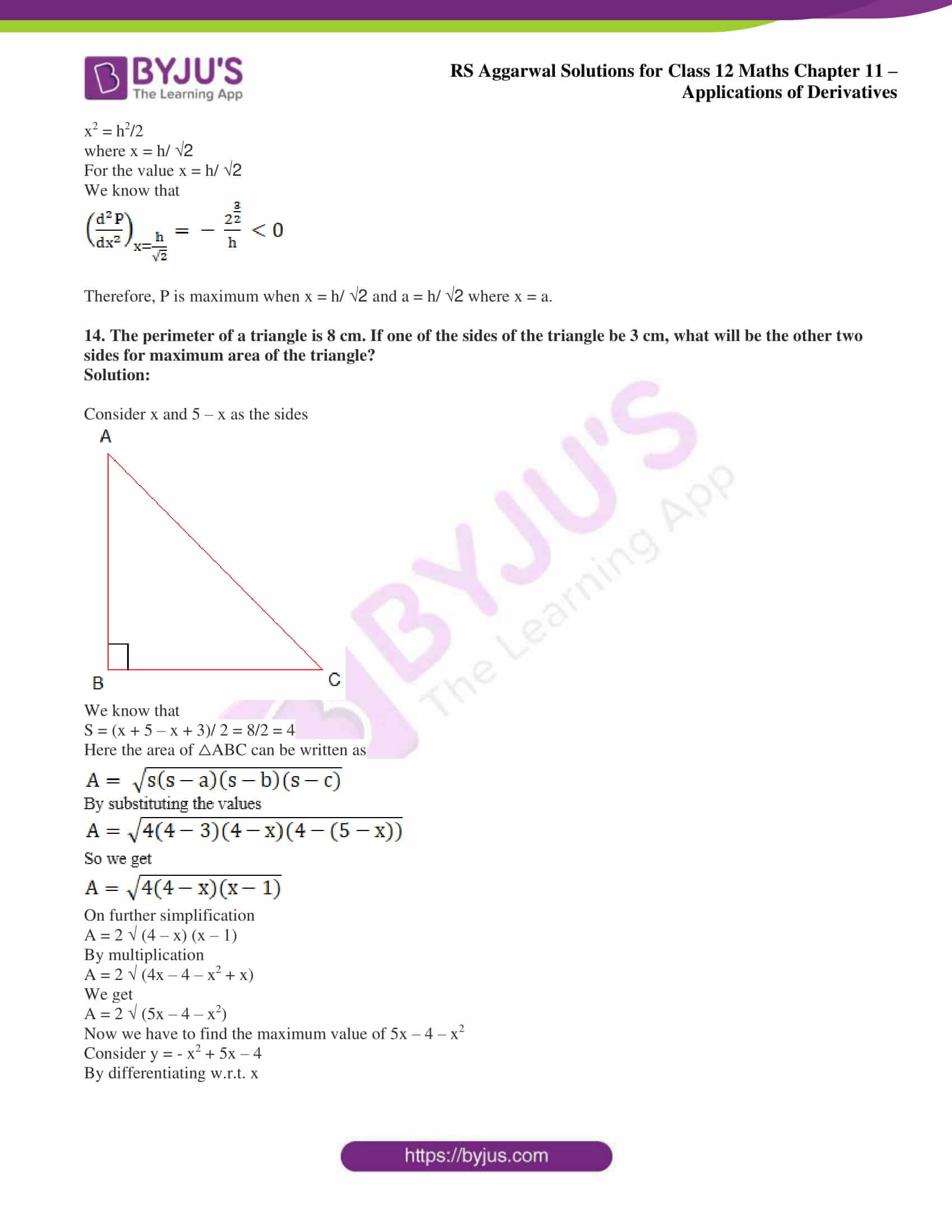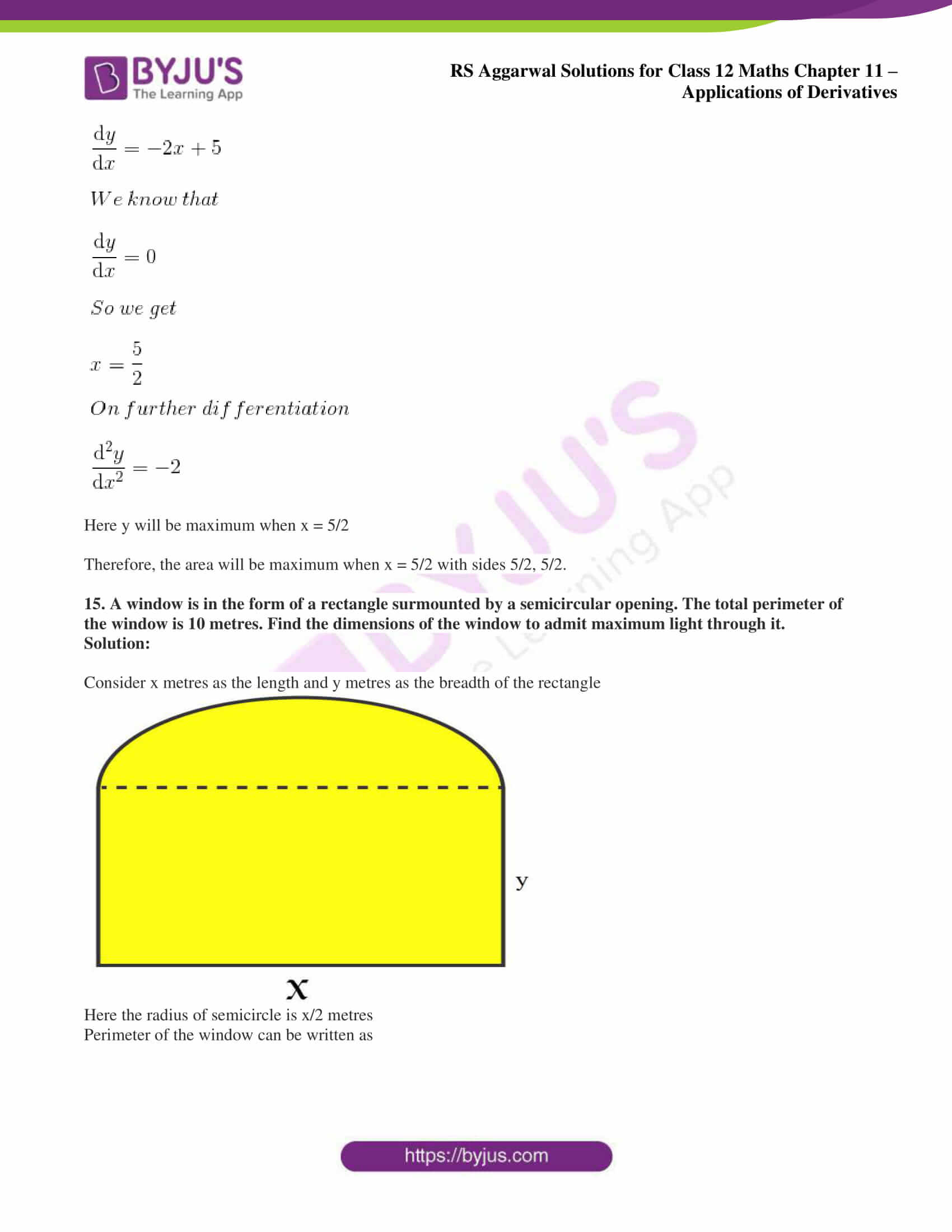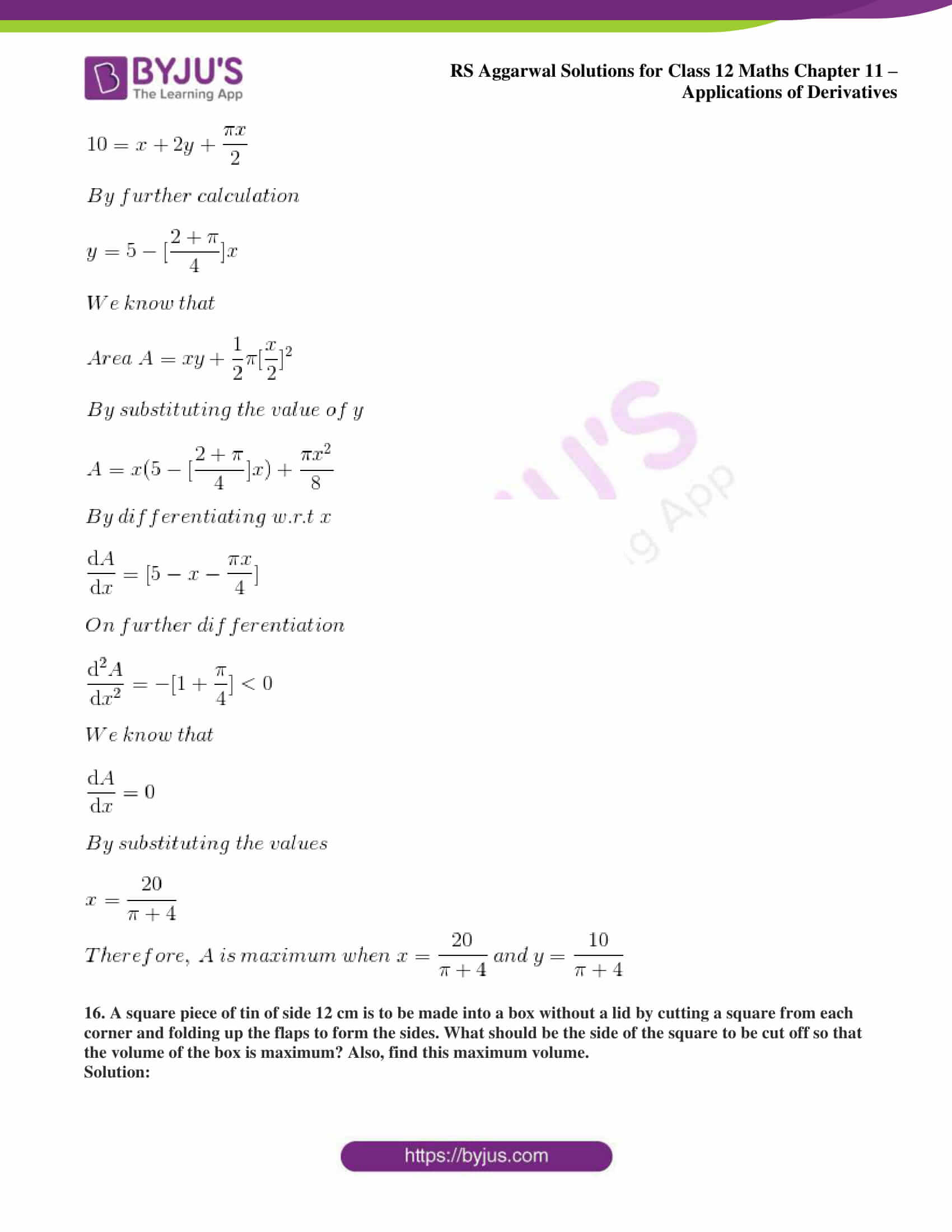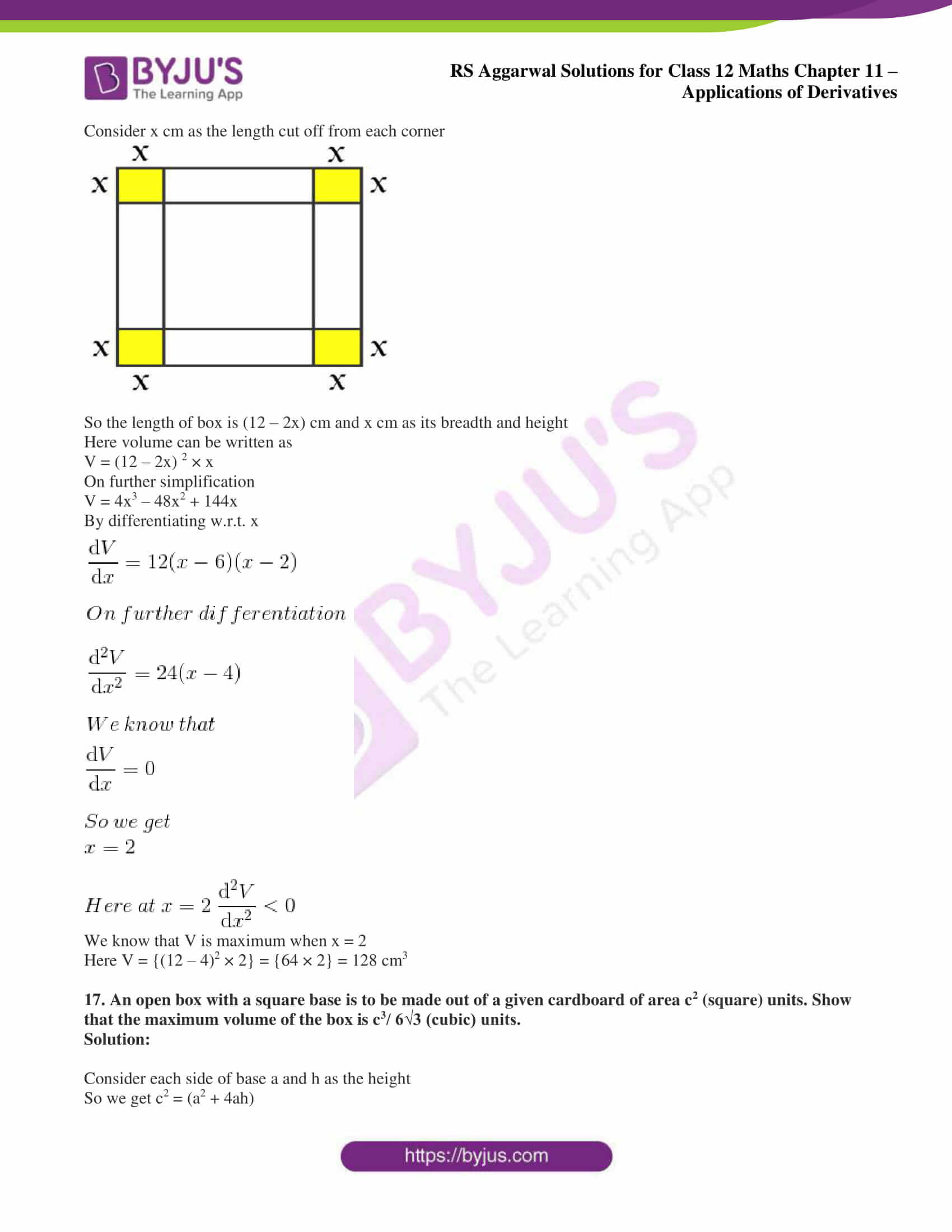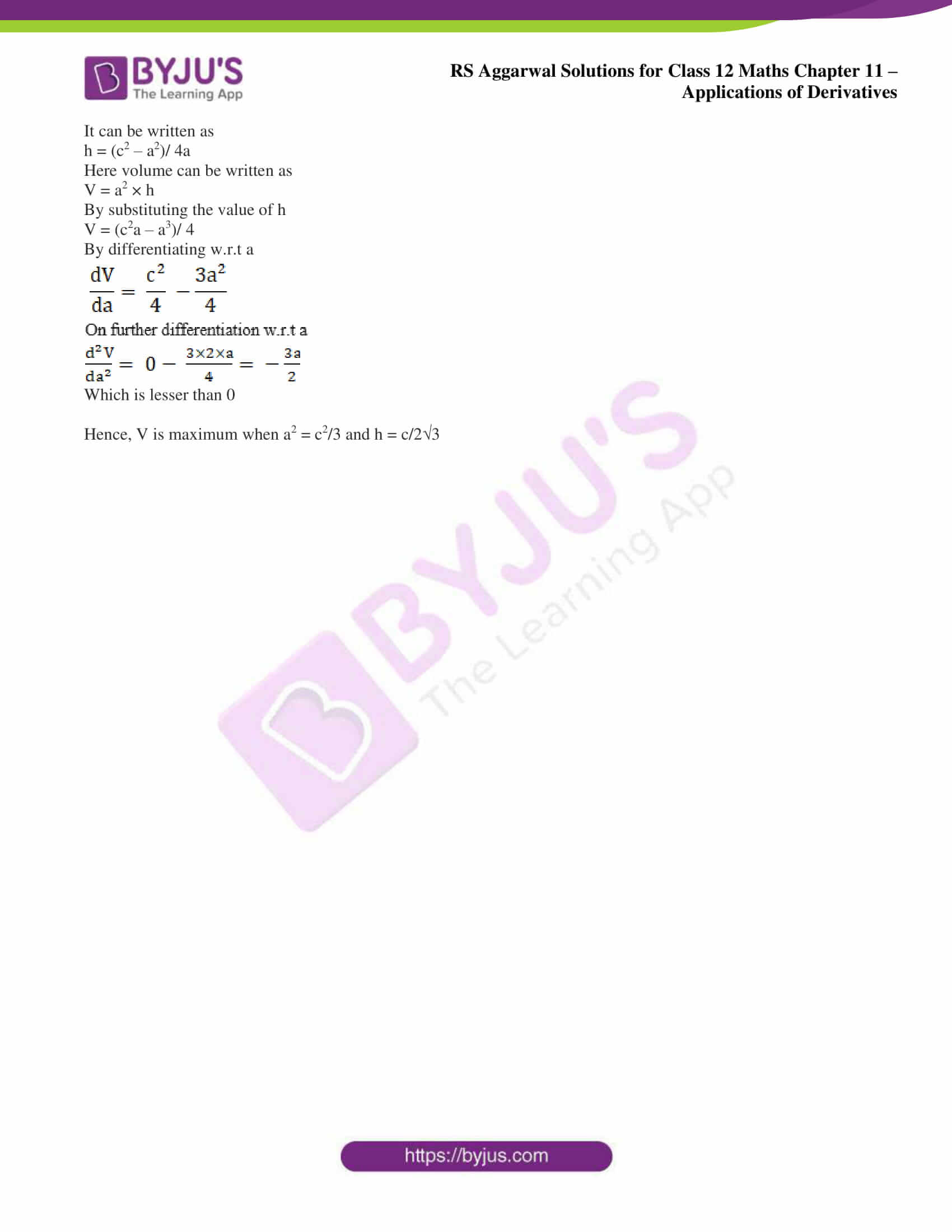### Access other exercise solutions of Class 12 Maths Chapter 11: Applications of Derivatives

Exercise 11A Solutions

Exercise 11B Solutions

Exercise 11C Solutions

Exercise 11D Solutions

Exercise 11E Solutions

Exercise 11G Solutions

Exercise 11H Solutions

### Access RS Aggarwal Solutions for Class 12 Maths Chapter 11: Applications of Derivatives Exercise 11F

1. Find two positive numbers whose product is 49 and the sum is minimum.

Solution:

Consider the two positive numbers as x and 49/x as their product is 49.

We know that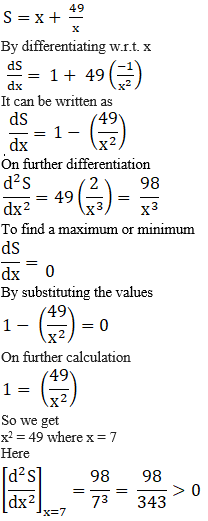Here x = 7 is a point of minimum and 7, 7 are the required numbers.

2. Find two positive numbers whose sum is 16 and the sum of whose squares is minimum.

Solution:

Consider the two positive numbers as x and 16 – x as their sum is 16.

We know that

S = x2 + (16 – x) 2

It can be written as

S = x2 + 256 – 32x + x2 = 2x2 – 32x + 256

By differentiating w.r.t. x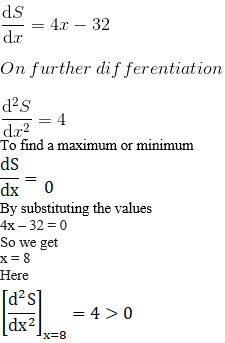x = 8 is the point of minimum and 8, 8 are the required numbers.

3. Divide 15 into two parts such that the square of one number multiplied with the cube of the other number is maximum.

Solution:

Consider the two parts as x and 15 – x

We know that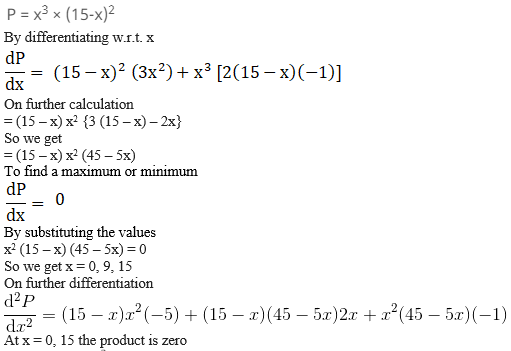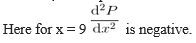When x = 9 the product is maximum.

Hence, 9, 6 are the required numbers.

4. Divide 8 into two positive parts such that the sum of the square of one and the cube of the other is minimum.

Solution:

Consider the two positive parts as x and 8 – x

We know that

S = x2 + (8 – x) 3

By differentiating w.r.t. x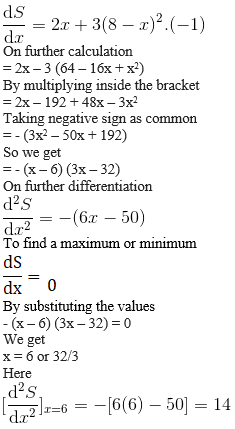Hence, x = 6 is a point of minimum and 6, 2 are the required numbers.

5. Divide a number into two parts such that the product of the pth power of one part and the qth power of the second part may be maximum.

Solution:

Consider the two parts of the number as x and a – x

We know that

y = xp (a – x) q

By differentiating w.r.t. x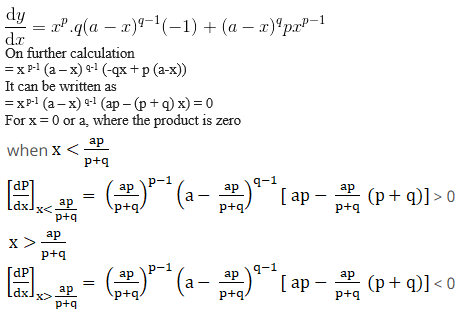Here, y is maximum for x = ap/ p + q

Hence, x = ap/ p + q and y = aq/ p + q are the two positive numbers required.

6. The rate of working of an engine is given by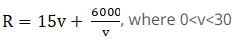and v is the speed of the engine. Show that R is the least when v = 20.

Solution:

It is given that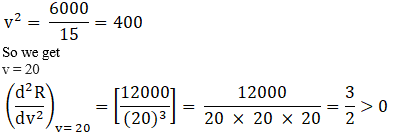Hence, v = 20 is a point of minimum.

7. Find the dimensions of the rectangle of area 96 cm2 whose perimeter is the least. Also, find the perimeter of the rectangle.

Solution:

Consider P as the fixed perimeter and x, y as the sides.

So area = xy = 96

It can be written as

y = 96/x

Similarly perimeter can be written as

P = 2 (x + y)

By substituting the value of y

P = 2 (x + 96/x)

By differentiating w.r.t. x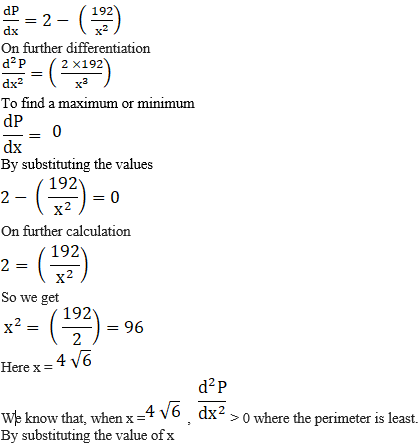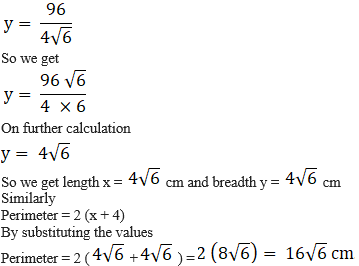8. Prove that the largest rectangle with a given perimeter is a square.

Solution:

Consider a as the fixed perimeter.

Take a rectangle having x and y as sides, a as perimeter and A as the area of the rectangle

So we get

2x + 2y = a

It can be written as

A = xy

Substituting the value of y

A = x [(a – 2x)/ 2]

By differentiating w.r.t. x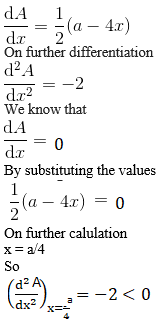We get

y = ½ (a – 2x)

It can be written as

y = ½ (a – a/2) = a/4

We know that x = y = a/4

Therefore, the rectangle is a square.

9. Given the perimeter of a rectangle, show that its diagonal is minimum when it is a square.

Solution:

Consider P as the fixed perimeter where x and y are the sides, P is the perimeter and D is the diagonal.

P = 2 (x + y)

It can be written as

y = ½ (P – 2x)

Here D2 = Z = x2 + y2

By further simplification

Z = x2 + ¼ (P – 2x) 2

By differentiating w.r.t. x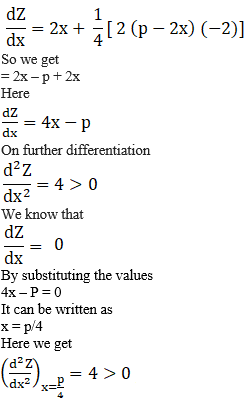Where the diagonal is minimum at x = p/4

Similarly

y = ½ (p – 2x)

By substituting the values

y = ½ (p – p/2) = p/4

We know that x = y = p/4

Therefore, the rectangle is a square.

10. Show that a rectangle of maximum perimeter which can be inscribed in a circle of radius a is a square of side √2 a.

Solution:

Consider ABCD as a rectangle which is inscribed in a circle having O as centre and a as radius

Now join OC where ∠COX = θ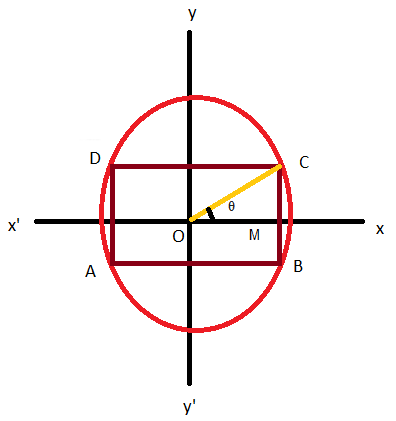So the co-ordinate of C are (a cos θ, a sin θ)

We get OM = a cos θ, MC = a sin θ

BC = 2MC = 2a sin θ and CD = 2OM = 2a cos θ

It can be written as

P = 2a (cos θ + sin θ)

By differentiating w.r.t. x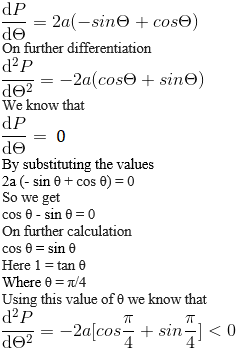Here, P is maximum at θ = π/4

BC = √2a = CD

11. The sum of the perimeters of a square and a circle is given. Show that the sum of their areas is least when the side of the square is equal to the diameter of the circle.

Solution:

Consider the side of square as x and radius of circle as r

It is given that

4x + 2 πr = k

We can write it as

x = (k – 2 πr)/ 4

We know that

A = x2 + πr2 = [(k – 2 πr)/ 4]2 + πr2

So we get

A = 1/16 (k2 – 4k πr + 4 π2 r2) + πr2

By differentiating w.r.t. r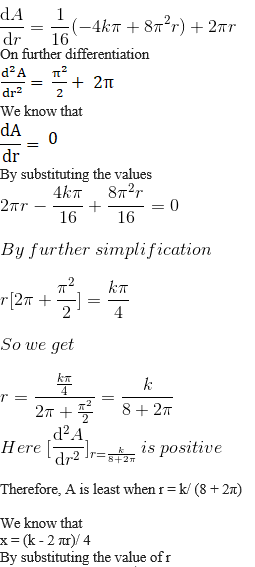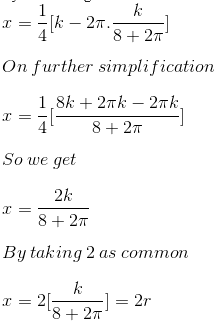Hence, A is least when side of square is equal to the diameter of the circle.

12. Show that the right triangle of maximum area that can be inscribed in a circle is an isosceles triangle.

Solution: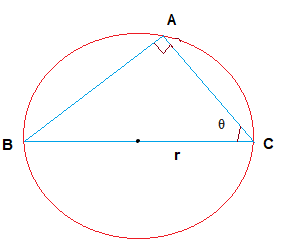We know that

AC = 2r cos θ

AB = 2r sin θ

It can be written as

△ABC = ½ × 2r cos θ × 2r sin θ

So we get

△ABC = r2 sin 2θ

Here the area is maximum when

sin 2θ = 1

So we get

2θ = 90o

Where θ = 45o

Therefore, △ABC is an isosceles triangle.

13. Prove that the perimeter of a right-angled triangle of given hypotenuse is maximum when the triangle is isosceles.

Solution:

Consider ABC as a right triangle with h as hypotenuse.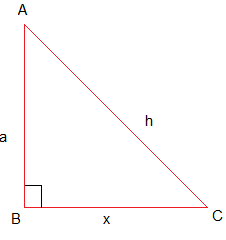Take BC = x as base and BA = a as altitude.

It can be written as

h2 = x2 + a2

By further simplification

a = (h2 – x2)

We know that

P = a + x + h

By substituting the value of a

P = (h2 – x2) + x + h

By differentiating w.r.t. x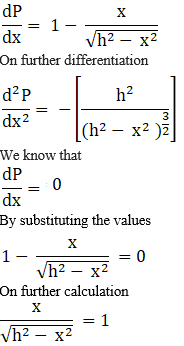So we get

x = (h2 – x2)

By squaring on both sides

x2 = h2 – x2

So we get

x2 = h2/2

where x = h/ 2

For the value x = h/ 2

We know that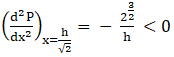Therefore, P is maximum when x = h/ 2 and a = h/ 2 where x = a.

14. The perimeter of a triangle is 8 cm. If one of the sides of the triangle be 3 cm, what will be the other two sides for maximum area of the triangle?

Solution:

Consider x and 5 – x as the sides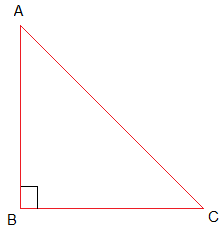We know that

S = (x + 5 – x + 3)/ 2 = 8/2 = 4

Here the area of △ABC can be written as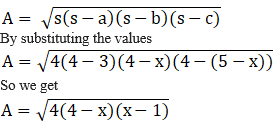On further simplification

A = 2 (4 – x) (x – 1)

By multiplication

A = 2 (4x – 4 – x2 + x)

We get

A = 2 (5x – 4 – x2)

Now we have to find the maximum value of 5x – 4 – x2

Consider y = – x2 + 5x – 4

By differentiating w.r.t. x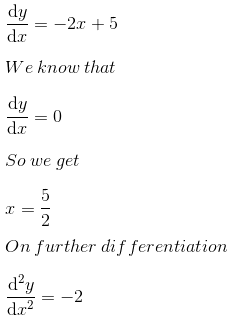Here y will be maximum when x = 5/2

Therefore, the area will be maximum when x = 5/2 with sides 5/2, 5/2.

15. A window is in the form of a rectangle surmounted by a semicircular opening. The total perimeter of the window is 10 metres. Find the dimensions of the window to admit maximum light through it.

Solution:

Consider x metres as the length and y metres as the breadth of the rectangle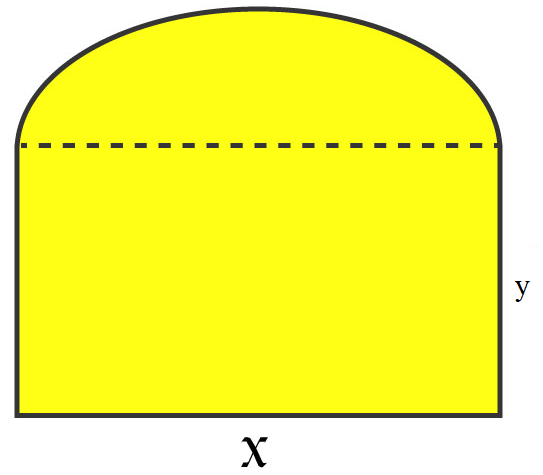Here the radius of semicircle is x/2 metres

Perimeter of the window can be written as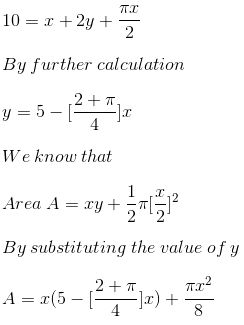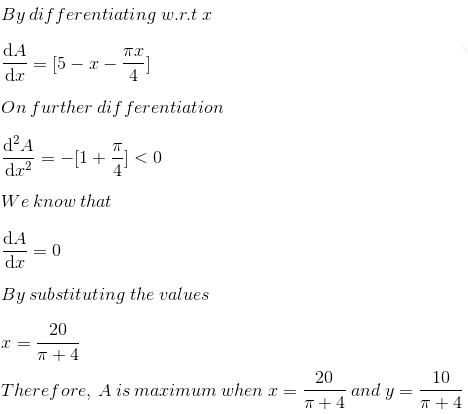16. A square piece of tin of side 12 cm is to be made into a box without a lid by cutting a square from each corner and folding up the flaps to form the sides. What should be the side of the square to be cut off so that the volume of the box is maximum? Also, find this maximum volume.

Solution:

Consider x cm as the length cut off from each corner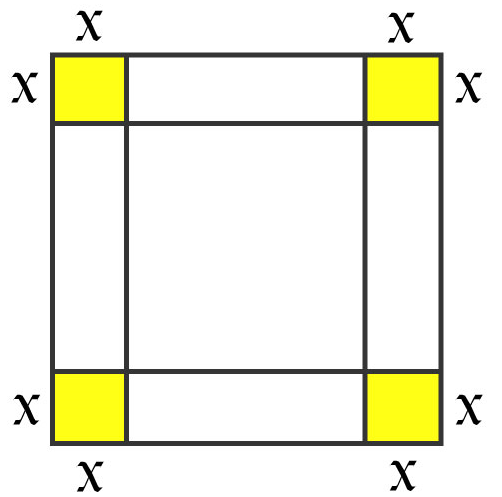So the length of box is (12 – 2x) cm and x cm as its breadth and height

Here volume can be written as

V = (12 – 2x) 2 × x

On further simplification

V = 4x3 – 48x2 + 144x

By differentiating w.r.t. x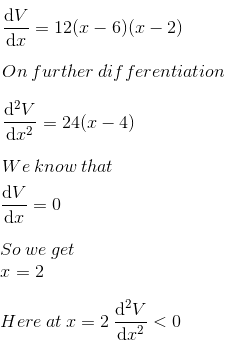We know that V is maximum when x = 2

Here V = {(12 – 4)2 × 2} = {64 × 2} = 128 cm3

17. An open box with a square base is to be made out of a given cardboard of area c2 (square) units. Show that the maximum volume of the box is c3/ 6√3 (cubic) units.

Solution:

Consider each side of base a and h as the height

So we get c2 = (a2 + 4ah)

It can be written as

h = (c2 – a2)/ 4a

Here volume can be written as

V = a2 × h

By substituting the value of h

V = (c2a – a3)/ 4

By differentiating w.r.t a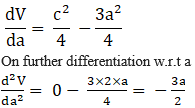Which is lesser than 0

Hence, V is maximum when a2 = c2/3 and h = c/23